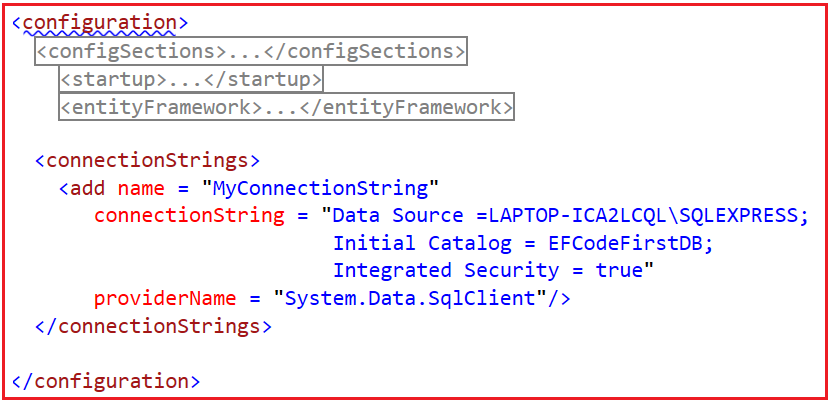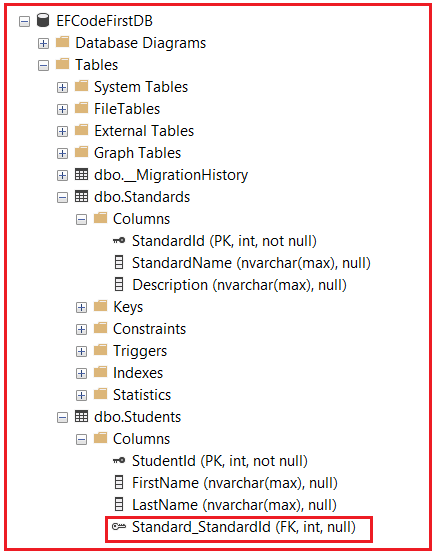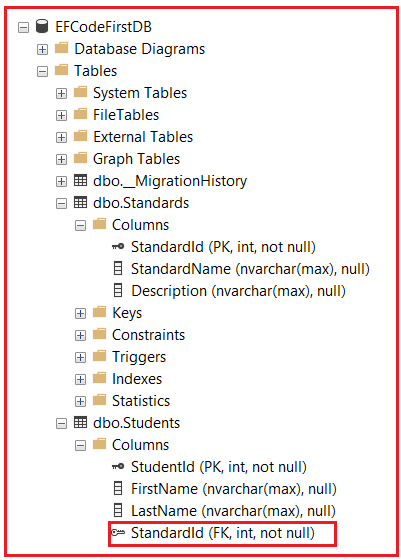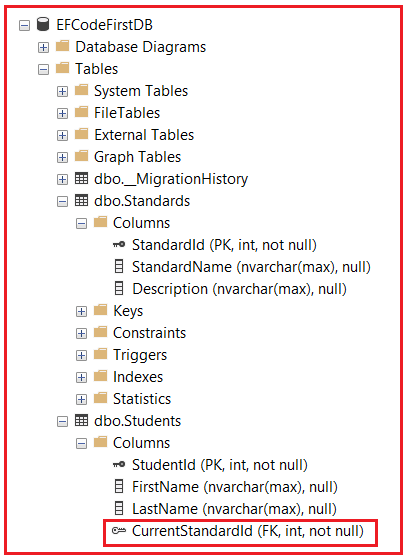# One-to-Many Relationships in Entity Framework Fluent API

## Configure One-to-Many Relationships using Entity Framework Fluent API

In this article, I am going to discuss how to configure One-to-Many Relationships between two Entities using Entity Framework Fluent API with Examples. Please read our previous article where we discussed how to configure One-to-Zero or One-to-One relationships between two entities. Let us understand this with an example. We are going to implement a One-to-Many relationship between the following Student and Standard Entities.

###### Student.cs

Create a class file with the name Student.cs and then copy and paste the following code into it.

```namespace EFCodeFirstDemo
{
public class Student
{
public int StudentId { get; set; }
public string FirstName { get; set; }
public string LastName { get; set; }
}
}
```
###### Standard.cs

Create a class file with the name Standard.cs and then copy and paste the following code into it.

```namespace EFCodeFirstDemo
{
public class Standard
{
public int StandardId { get; set; }
public string StandardName { get; set; }
public string Description { get; set; }
}
}
```

In Entity Framework Code First Approach, we can configure the one-to-many relationship between two entities in the following ways.

1. By following Conventions
2. By using Fluent API Configurations

#### By Following Conventions:

There are certain conventions in Entity Framework which if followed in entity classes (domain classes), then it will automatically implement one-to-many relationships between the two tables in the database. In this case, we do not need to configure anything else. Let us understand all the conventions which create one-to-many relationships in Entity Framework.

##### Convention 1 for Implementing One-to-Many Relationship

Our Requirement is to establish a One-to-Many Relationship between the Student and Standard entities. That means one can student can be associated with one standard and one standard can be assigned to many students. We can achieve this very easily by including a Reference Navigation Property of type Standard in the Student entity class. So, modify the Student entity as follows to include the Standard Reference Navigation Property.

```namespace EFCodeFirstDemo
{
public class Student
{
public int StudentId { get; set; }
public string FirstName { get; set; }
public string LastName { get; set; }
public Standard Standard { get; set; }
}
}
```

As you can see in the above class, the Student Entity includes a Reference Navigation Property of Standard type. So, there can be many students in a single standard. This will result in a One-to-Many relationship between the Students and Standards table in the database, where the Students table includes the foreign key Standard_StandardId.

Please make sure to have the connection string with the name MyConnectionString within the app.config file or web.config file as shown in the below image.Next, modify the context class as follows.

```using System.Data.Entity;
namespace EFCodeFirstDemo
{
public class EFCodeFirstContext : DbContext
{
public EFCodeFirstContext() : base("name=MyConnectionString")
{
//Setting the Database Initializer as DropCreateDatabaseAlways
Database.SetInitializer(new DropCreateDatabaseAlways<EFCodeFirstContext>());
}

public DbSet<Student> Students { get; set; }
public DbSet<Standard> Standards { get; set; }
}
}
```

Next, modify the Main method of the Program class as follows.

```using System;
namespace EFCodeFirstDemo
{
class Program
{
static void Main(string[] args)
{
using (EFCodeFirstContext context = new EFCodeFirstContext())
{
var standard = new Standard() { StandardId = 1, Description = "Standard-1"};
var student = new Student() {FirstName = "Pranaya", LastName = "Rout"};
context.SaveChanges();
}
}
}
}
```

With the above changes in place, run the application and verify the database and you should see the following.##### Convention 2 for Implementing One-to-Many Relationship

Another way to implement a One-to-Many Relationship using convention is to include a collection navigation property in the principal entity. Here, the Standard is the Principal Entity and Student is the dependent entity. So, we need to include the collection navigation property Students within the Standard Entity. So, modify the Standard Entity as follows.

```using System.Collections.Generic;
namespace EFCodeFirstDemo
{
public class Standard
{
public int StandardId { get; set; }
public string StandardName { get; set; }
public string Description { get; set; }
public ICollection<Student> Students { get; set; }
}
}
```

In the above example, we have included a collection navigation property of type ICollection<Student> within the Standard Principal Entity. This will also automatically result in a one-to-many relationship between the Student and Standard entities. Next, we need to remove the Standard Reference Navigation Property from the Student entity. So, modify the Student Entity as follows:

```namespace EFCodeFirstDemo
{
public class Student
{
public int StudentId { get; set; }
public string FirstName { get; set; }
public string LastName { get; set; }
}
}
```

With the above changes in place, run the application and see the database table. This will result same as the previous convention as shown in the below image.##### Convention 3 for Implementing One-to-Many Relationship

It is also possible to implement a One-to-Many relationship between two entities by including the navigation properties at both ends.

Modify the Student Entity as follows:

```namespace EFCodeFirstDemo
{
public class Student
{
public int StudentId { get; set; }
public string FirstName { get; set; }
public string LastName { get; set; }
public Standard Standard { get; set; }
}
}
```

Modify the Standard Entity as follows:

```using System.Collections.Generic;
namespace EFCodeFirstDemo
{
public class Standard
{
public int StandardId { get; set; }
public string StandardName { get; set; }
public string Description { get; set; }
public ICollection<Student> Students { get; set; }
}
}
```

As you can see in the above two classes, the Student entity includes a reference navigation property of the Standard type and the Standard entity includes a collection navigation property of the ICollection<Student> type which results in one-to-many relationships between Students and Standards tables in the database. This implementation also produces the same result as the previous two examples.

##### Convention 4 for Implementing One-to-Many Relationship

So far the examples we have discussed, the Entity Framework creates the foreign key with the default name i.e. Standard_StandardId. It is also possible to provide a user-defined name for the foreign key. To do so, we need to include the foreign key property StandardId along with its reference navigation property Standard within the Student entity. So, modify the Student entity class as follows.

```namespace EFCodeFirstDemo
{
public class Student
{
public int StudentId { get; set; }
public string FirstName { get; set; }
public string LastName { get; set; }
public int StandardId { get; set; }
public Standard Standard { get; set; }
}
}
```

Next, modify the Main method of the Program class as follows:

```using System;
namespace EFCodeFirstDemo
{
class Program
{
static void Main(string[] args)
{
using (EFCodeFirstContext context = new EFCodeFirstContext())
{
var standard = new Standard() { StandardId = 1, Description = "Standard-1"};
context.SaveChanges();

var student = new Student() {FirstName = "Pranaya", LastName = "Rout", StandardId = 1};
context.SaveChanges();
}
}
}
}
```

With the above changes in place, run the application and see the database table. In this case, it will create the foreign key column with the name StandardId as shown in the below image.##### How to Configure One-to-Many Relationships using Fluent API in Entity Framework?

Generally, we do not need to configure the one-to-many relationship in the entity framework because one-to-many relationship conventions cover all combinations. However, you may configure relationships using Fluent API in one place to make them more maintainable. Consider the following Student and Standard entity classes.

Modify the Student Entity as follows:

```namespace EFCodeFirstDemo
{
public class Student
{
public int StudentId { get; set; }
public string FirstName { get; set; }
public string LastName { get; set; }
public int CurrentStandardId { get; set; }
public Standard CurrentStandard { get; set; }
}
}```

Modify the Standard Entity as follows:

```using System.Collections.Generic;
namespace EFCodeFirstDemo
{
public class Standard
{
public int StandardId { get; set; }
public string StandardName { get; set; }
public string Description { get; set; }
public ICollection<Student> Students { get; set; }
}
}
```

Now, you can configure the one-to-many relationship for the above two entities using Entity Framework Fluent API by overriding the OnModelCreating method in the context class. To do so, we are going to use the following three Fluent API methods.

1. HasRequired(): It configures a required relationship from the entity type. Instances of the entity type will not be able to be saved to the database unless this relationship is specified. The foreign key in the database will be non-nullable.
2. WithMany(): It configures the relationship to be required:many with a navigation property on the other side of the relationship.
3. HasForeignKey(): It configures the relationship to use foreign key property(s) that are exposed in the object model.

Now, modify the context class as follows:

```using System.Data.Entity;
namespace EFCodeFirstDemo
{
public class EFCodeFirstContext : DbContext
{
public EFCodeFirstContext() : base("name=MyConnectionString")
{
//Setting the Database Initializer as DropCreateDatabaseAlways
Database.SetInitializer(new DropCreateDatabaseAlways<EFCodeFirstContext>());
}

protected override void OnModelCreating(DbModelBuilder modelBuilder)
{
//Configures One-to-Many Relationship
modelBuilder.Entity<Student>()
.HasRequired<Standard>(s => s.CurrentStandard)
.WithMany(std => std.Students)
.HasForeignKey<int>(s => s.CurrentStandardId);
}

public DbSet<Student> Students { get; set; }
public DbSet<Standard> Standards { get; set; }
}
}
```
##### Understanding the OnModelCreating Method code.

Let us understand the OnModelCreating Method code step by step.

1. First, we need to start configuring with one of the entity classes. So, modelBuilder.Entity<student>() starts with the Student entity.
2. Then, HasRequired<Standard>(s => s.CurrentStandard) method specifies that the Student entity has required the CurrentStandard property. This will create a NotNull foreign key column in the Students Database table.
3. Then, WithMany(std => std.Students) specifies that the Standard entity class includes many Student entities. Here, many are represented by the ICollection type property.
4. Now, if the Student entity does not follow the Id or Entity+Id property convention for the foreign key, then we can specify the name of the foreign key using the HasForeignKey method. The HasForeignKey<int>(s => s.CurrentStandardId); specifies the foreign key property in the Student entity.

Next, modify the Main method of the Program class as follows:

```using System;
namespace EFCodeFirstDemo
{
class Program
{
static void Main(string[] args)
{
using (EFCodeFirstContext context = new EFCodeFirstContext())
{
var standard = new Standard() { StandardId = 1, Description = "Standard-1"};
context.SaveChanges();

var student = new Student() {FirstName = "Pranaya", LastName = "Rout", CurrentStandardId  = 1};
context.SaveChanges();
}
}
}
}
```

With the above changes in place, run the application and you should see the database tables as expected as shown in the below image.##### How to Configure Cascade Delete using Fluent API?

Cascade delete means automatically deleting the child rows when the related parent row is deleted. For example, if Standard is deleted from the Standard table, then automatically all the students belonging to that should also be deleted from the Students table. To implement this, we need to use WillCascadeOnDelete Fluent API Method. Modify the context class as follows.

```using System.Data.Entity;
namespace EFCodeFirstDemo
{
public class EFCodeFirstContext : DbContext
{
public EFCodeFirstContext() : base("name=MyConnectionString")
{
//Setting the Database Initializer as DropCreateDatabaseAlways
Database.SetInitializer(new DropCreateDatabaseAlways<EFCodeFirstContext>());
}

protected override void OnModelCreating(DbModelBuilder modelBuilder)
{
//Configures One-to-Many Relationship
modelBuilder.Entity<Student>()
.HasRequired<Standard>(s => s.CurrentStandard)
.WithMany(std => std.Students)
.HasForeignKey<int>(s => s.CurrentStandardId)